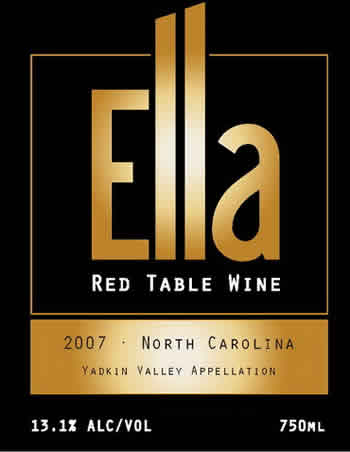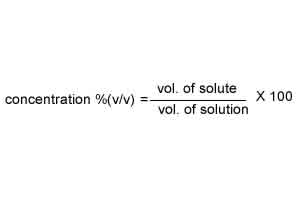Concentration(v/v) There are many ways to express the concentration of a solution. A bottle of wine has the label 13.1% (v/v). That means that 13.1 ml of alcohol are present in every 100 ml of wine. The label on the right states that the wine contains 13.1% (v/v) alcohol (ethanol) where (v/v) is volume per volume. This is known as percentage by volume and gives the volume of the solute present in 100 ml of solution.120.0 ml of wine contains 20.0 ml of ethanol. What is the concentration (v/v) of ethanol in this solution? SolutionWhat volume of a 15.0% (v/v) alcohol solution will contain exactly 10.0 ml of alcohol? Solution 200.0 ml of a 15.0% (v/v) hydrochloric acid solution will contain what volume of acid? Solution Continue